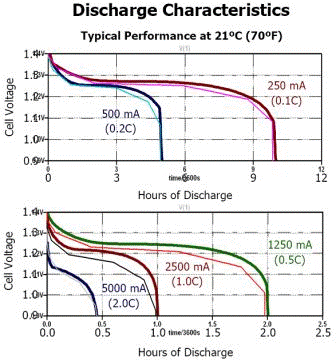# Modelling a Ni-MH battery with hints on Li-Ion battery modeling

Here is a model for a NiMH cell using one of LTspice's many great unique features (its nonlinear capacitor) to use a simple table to model the cell voltage verses charge characteristic. Internal resistance verses state of charge was also modeled using another table.

If you have the discharge curves for your Li-Ion cell, it's likely you could modify the HiMH model's tables to match your cell's characteristics.

Application information on the nonlinear is in the following chapter in Help: LTspice -> Circuit Elements -> Capacitor
__________________________________________________________

There is also a general nonlinear capacitor available. Instead of specifying the capacitance, one writes an expression for the charge.

LTspice will compile this expression and symbolically differentiate it with respect to all the variables, finding the partial derivative's that correspond to capacitances.

Syntax: Cnnn n1 n2 Q=<expression>; [ic=<value>] [m=<value>]

There is a special variable, x, that means the voltage across the device. Therefore, a 100pF constant capacitance can be written as

Cnnn n1 n2 Q=100p*x

A capacitance with an abrupt change from 100p to 300p at zero volts can be written as

Cnnn n1 n2 Q=x*if(x<0,100p,300p)

This device is useful for rapidly evaluating the behavior of a new a hypothetical charge model for, e.g., a transistor.
__________________________________________________________

Here is my simple HiMH sub circuit:

.subckt NH15-2.5 A K
C1 A K 15m Rser=18m ; ac impedance
R1 A 1 R= tbl(V(1,K),1,150m,1.1,70m,1.2,50m,1.26,40m,1.27,30m)
C2 1 K= tbl(x,0,0,1.1,108,1.2,1k08,1.26,3k6,1.27,7k2,1.34,8k64,1.4,9k)
D1 K 1 1v5 ; self discharge, reverse and over voltages
.model 1v5 d(Vrev=1.5 Ron=0.1 Roff=1k2)
.ends NH15-2.5

And here is direct link an LTspice .asc test file: File:NHcell.asc
__________________________________________________________

Model vs. Datasheet
<img src="">